# How to count colored cells in Excel?

You might be happy after knowing that there would be several methods in Excel that help the users to count the colored cells. Yes, Excel offers inbuilt functions using which you can count colored cells.

An Excel worksheet may contain formatting in it for analysis purposes. It consists of some colored and simple rows. Excel enables multiple ways to count the colored cell containing by an Excel spreadsheet. You can learn all of them very easily and use the one which you found more suitable.

This chapter will guide each step in detail to calculate the colored cells or rows in an Excel worksheet.

## Methods to count colored cell

In this chapter, we will discuss three different methods to count the colored cells in Excel.

On other websites, you may get more methods as well. Here, we have chosen the easiest one.

## Method 1: Using GET.CELL function

GET.CELL is a special type of function of Excel that can be used to count the colored cells. It is basically a Macro4 function. It does not work like regular functions as others in Excel.

GET.CELL function works on name ranges that are used to create a color range to get the color code. Later that color code helps to find the number of colored cells.

### Steps to count colored cells using GET.CELL function

Follow the instructions to count the colored cells in an Excel worksheet. It usually requires three most important steps to get the result.

1. Firstly, create a named range using the GET.CELL function.
2. Now, use that named range to get the color code.
3. With the help of the color number (code), count the total number of colored cells.

Let’s see with an example to understand how these steps will get performed.

### Example 1

Step 1: We have a worksheet containing some simple and some colored cells.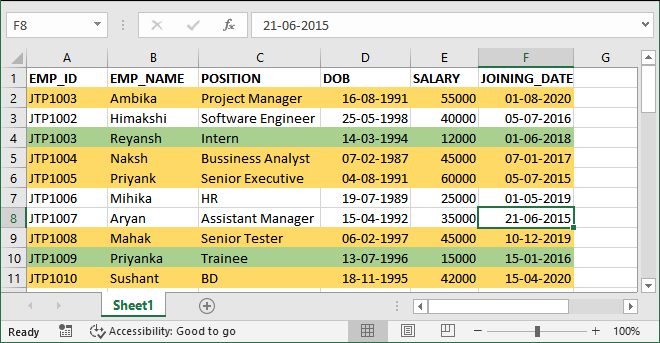Now, we will apply those three steps in detail.

### Creating color range

Step 2: Go to the Formulas tab and click on the Define Name option in the ribbon.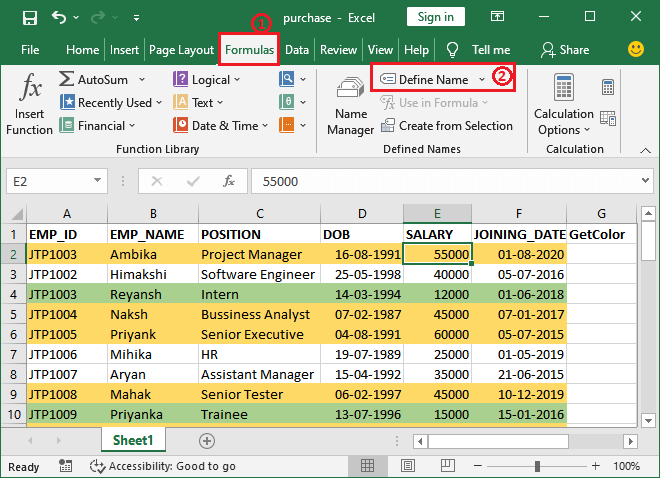Step 3: A dialog box will open, where enter the following details in it and click OK:

Name: GetColor

Scope: Choose the scope as Workbook.

Refers to: =GET.CELL(38, Sheet1!\$A2)In the Refers to field, we have used GET.CELL() formula where first parameter value is 38, which is a parameter code. Here, 38 indicates to the info type. It means which type of information you want from GET.CELL method. Here, 38 refers to the background color information I want to get.

The second parameter value is Sheet1!\$A2, from which you want to extract the information. You can define the reference of the column containing the colored cell inside them.

### Getting the color code for each cell

Now, we will get the color code for each row individually and store them in a new column.

Step 4: Use the following formula =GetColor in the adjacent cell of each row and press the Enter key.It will return a specific number if the cell contains the background color. Otherwise, it will return 0.

Step 5: Look in the screenshot below that a number code – 36 has returned for the cell containing Yellow color.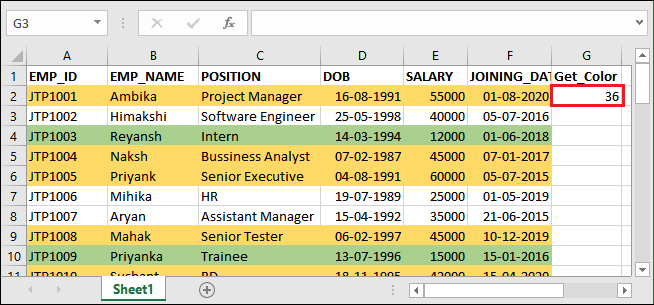Let’s see this formula for the first three cells having yellow, green, and no color. Apply the same =GetColor formula in all the respective rows.

Step 6: For the next row number, GetColor has returned 0 as it does not contain any background color.Step 7: For the row colored with green background color, GetColor has returned 50 as its color code number.Similarly, find the color code for all the rows one by one and move to the last step.

Step 8: See the worksheet after finding the color code for all rows containing some data.### Count colored cell using color codes

The final step is to count the total number of cells having any background color. So, move forward.

Step 9: Create two more cells below the dataset and colored them with the same color that your worksheet already containing. See as showing below.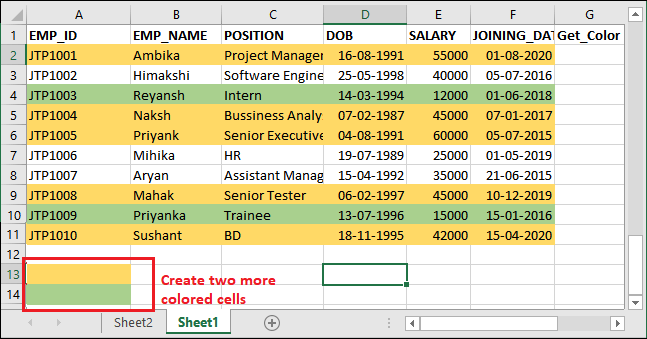Step 10: Now, in the adjacent row of the newly create cells, use the following COUNTIF() formula.

=COUNTIF(G2:G11,GetColor)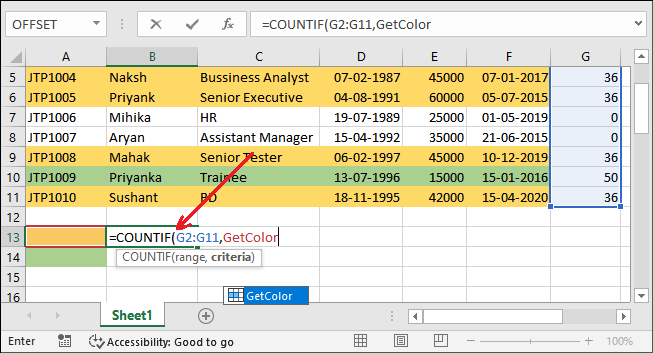Step 11: Hit the Enter key and see the result returned by it. Note that – it has returned 5 for yellow color cells.Five rows are colored with yellow color in this Excel sheet.

Step 12: Now, apply the same formula in the adjacent row of the green color cell.

=COUNTIF(G2:G11,GetColor)Step 13: See the result returned for the green color row in the below output, i.e., 2. Means two rows are colored with green color.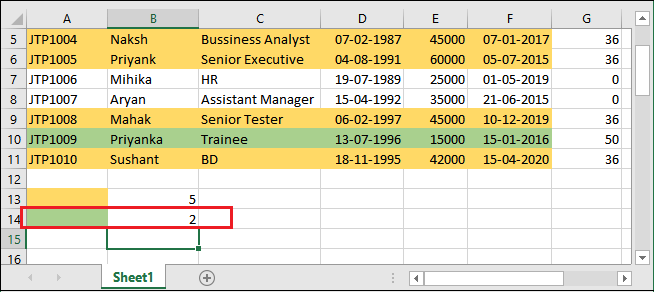Two rows are colored with green color in this Excel sheet

Conclusion: 5 yellow color rows and 2 green color rows. Hence, this worksheet is containing total 7 colored rows.

Now, let’s see the next method!

## Method 2: Using Filter and Subtotal function

The second method to count the colored cells in the Excel worksheet is – Filter and Subtotal, which are inbuilt functions of Excel. We will show you the steps to use them. This method requires only three steps to count the colored cell in an Excel worksheet using the Filter and Subtotal functions.

Steps to count colored cells

1. Firstly, use the subtotal() function to count all visible cells within a range.
2. Then, apply the filter on header and sort the rows of different colors.
3. The subtotal value will automatically change to a new value for currently visible colored cells after filtering the cells. Find the subtotal for each color and add them to get the total count of colored cells.

Example 2

We will apply these steps to the following dataset containing some colored and normal cells.In this worksheet, two colors are used: Yellow and Green.

Step 1: Select any cell below the dataset and write the following formula inside it.

=SUBTOTAL(102,F2:F11)

Here, 102 refers to the COUNT() function and F2:F11 is the range of cells.Step 2: See the result calculated by the SUBTOTAL() function for this dataset.Step 3: Now, select the header (user-defined heading, i.e., column A) of the worksheet and navigate to the Data tab.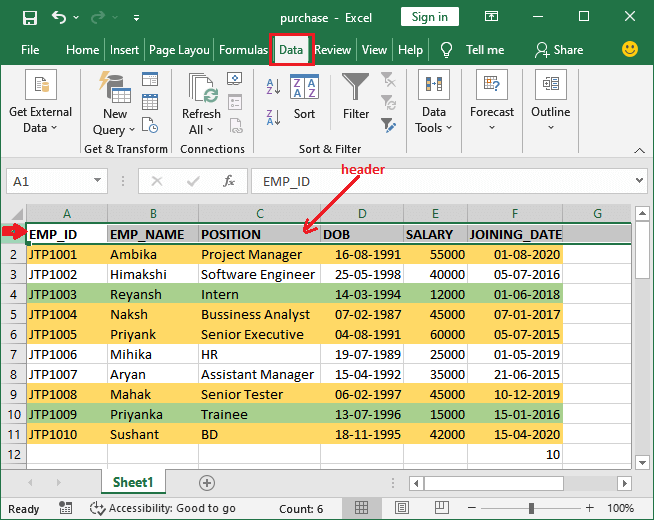Step 4: Inside the Sort & Filter section, click the Filter option in the Excel ribbon that will apply the filter on all headers.A dropdown type button will add to each cell of the header, as showing below:Step 5: Click any of these filter dropdown buttons, e.g., Salary dropdown button. A list will open where click the Sort by color in the list.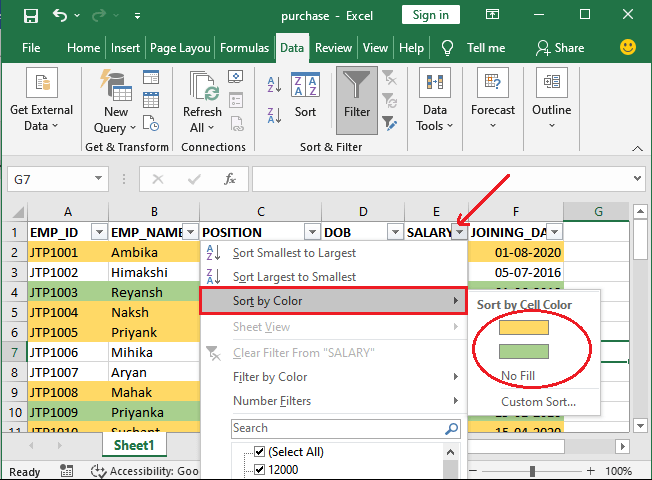Since there are two colors used to highlight the cell in the dataset. So, those two are showing here to sort the data.

Step 6: Select one color to select the data and see the updated value of the SUBTOTAL() result (calculated earlier) with sorted data.

First, we will choose Green color and see the total green color cells, i.e., 2.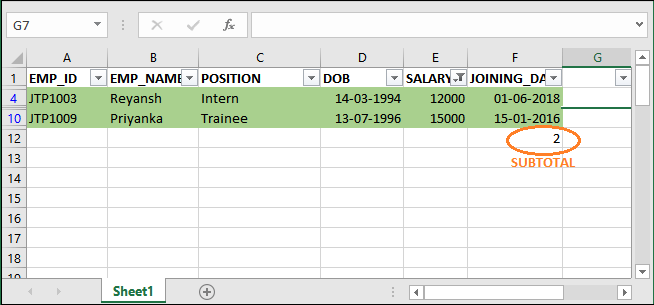Then, we will choose Yellow color and see the total green color cells, i.e., 5.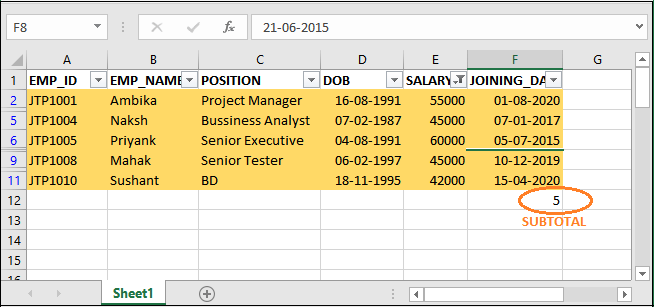Get the sum of both results (5+2=7). This means the total seven cells are colored in this Excel sheet. So, using this way, you can count the number of colored rows in an Excel worksheet.

## Method 3: Using VBA code

The above two methods are performed on Excel data using the in-built method of Excel. Now, we will count the colored cells by writing the code on VBA. This method is the easiest one for those who are comfortable in writing code and fine to use VBA.

In the above two methods, we have used in-built functions. Using VBA, we can now create a custom function to count the colored cells directly. It will work similar to the COUNTIF method. See how it will be done:

### VBA Code

Here, the first line of the code is countColoredCells, which is the function name. This code is a user-defined function (UDF), not a SUB Procedure to run. You can also copy the code from here and write it to your own code and run it to count the colored cells.

### Parameters for this function

This user-defined function will take two arguments.

Color – Color that we need to count. It means to provide the reference of the cell containing that color, e.g., F2.

Range – Cell range within which we want to count the cells colored with the specified color, e.g., D2:D15.

### Syntax

The following will be the syntax for this created function –

### What does it return?

This custom function will return the total number of cells having any specific color in its background.

### Steps to VBA editor in Excel

These are some steps to go to Excel VBA editor and write the code in it.

Step 1: On your active Excel workbook, press the Alt+F11 shortcut key to open the VBA code editor, like this.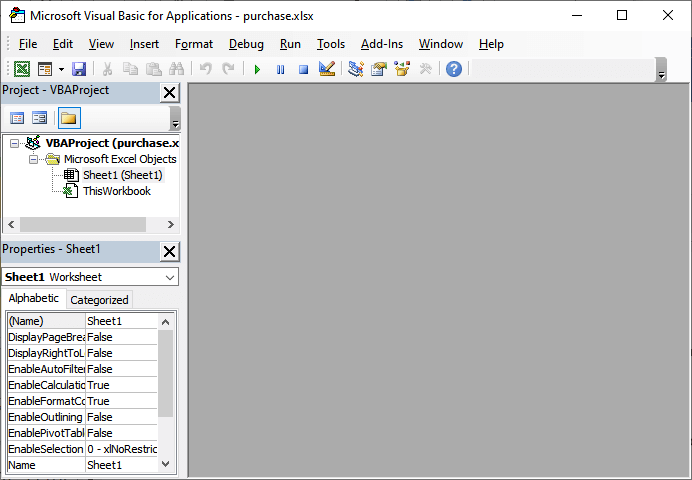Step 2: Here, navigate to the Insert -> Module in the menu bar that will insert a new module to write the code.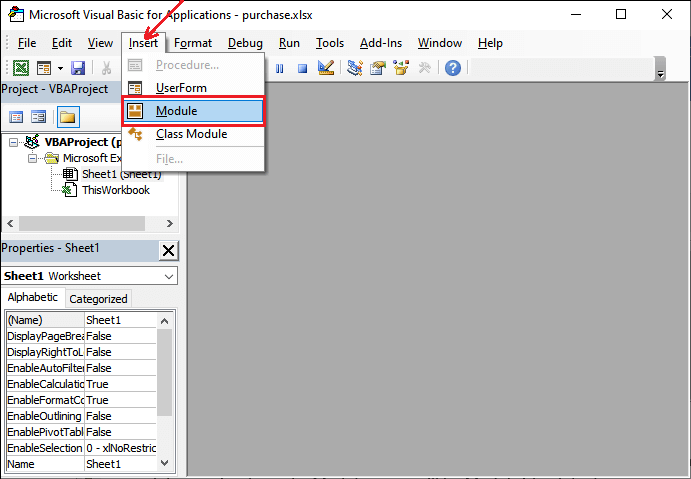A module named Module1 (default) will add to the currently active workbook in the VBA code editor.Step 3: Copy the above user-defined code and paste it into this new module window.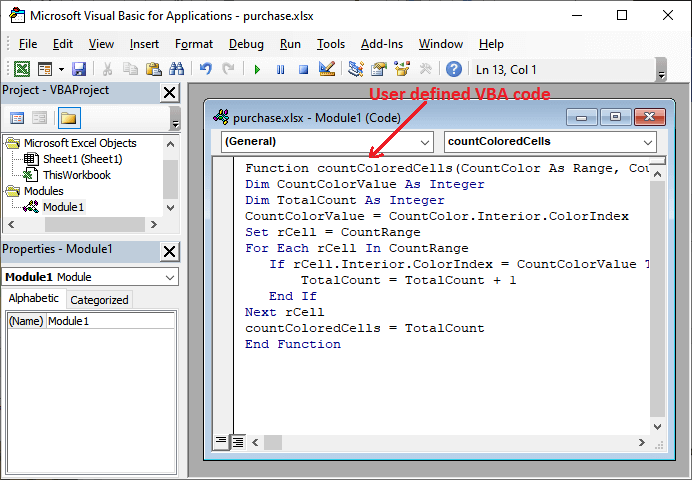Now, the countColoredCells() function has been added to this worksheet.

#### Note: You don’t need to save the VBA code immediately. Currently, you just minimize the VBA code editor panel and follow further steps.

Step 4: Since your worksheet contains two colors. So, create two cells in a new column and colored them with those colors, as showing below.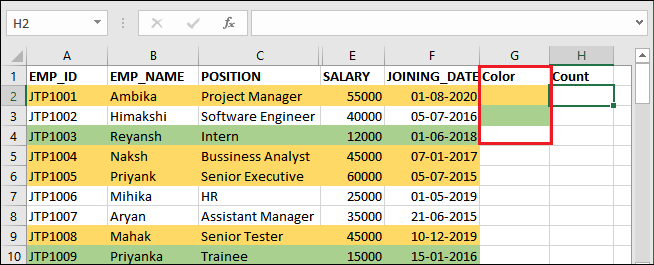Step 5: Now, use the created function countColoredCells firstly in H2 cell to count the total number of cells colored by yellow color.

=countColoredCells(G2,F\$2:F\$11)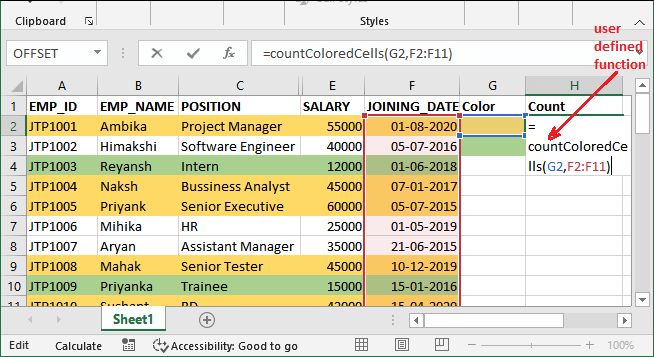Step 6: Press the Enter key to get the calculated result. It will return the number of cells colored with your selected color.See it has returned value 5, which means that the five rows are colored with yellow color within the selected range.

Step 7: Now, we will count the number of cells colored with Green color using the following countColoredCells formula. This time select G3 cell for green color and write this formula in H3 cell.

=countColoredCells(G3,F\$2:F\$11)Step 8: Hit the Enter key to get the calculated result and see the result returned by it, i.e., 2. This means that 2 cells are colored with Green color within the selected range.Step 9: Sum both results to get the total. Write the following addition formula in the H4 cell:

=H2+H3 and press the Enter key.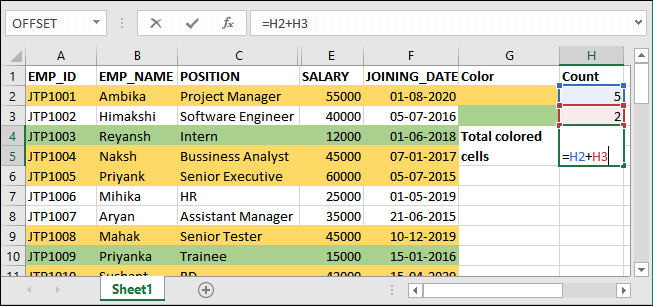Step 10: Total colored cells in this worksheet are 7.Now, close the VB editor. VBA code is a feature of the macro. So, this feature cannot be saved in a normal Excel file (macro-free workbook). When you save it normally, a popup will display to you.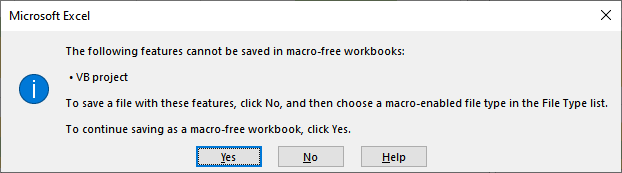To save this file, click No and choose the file type as Macro-enabled inside the File Type List and save it again.